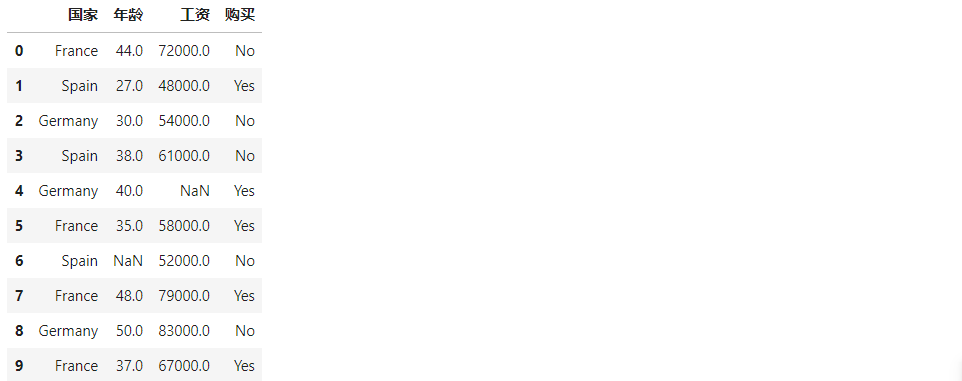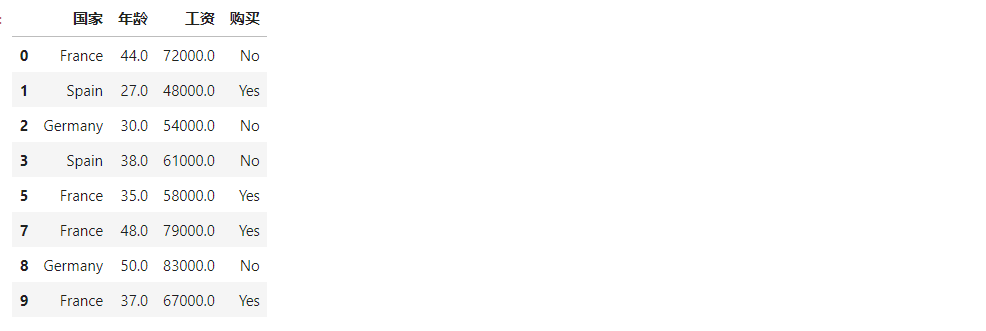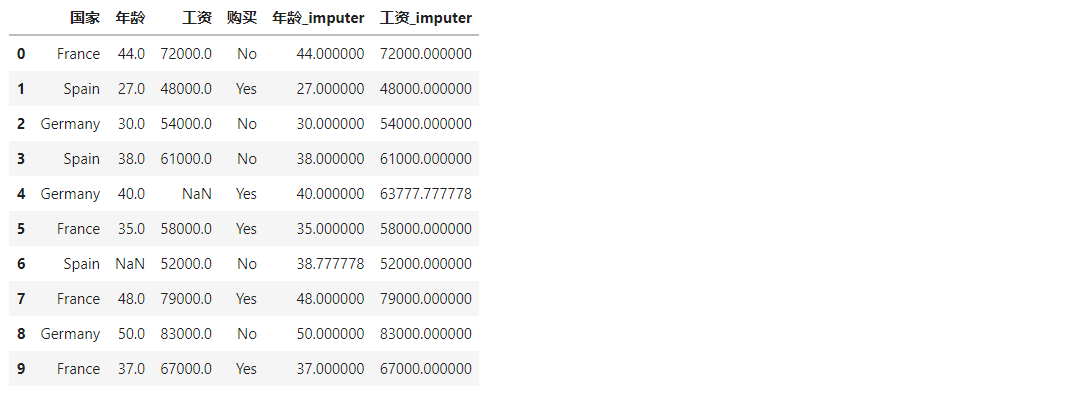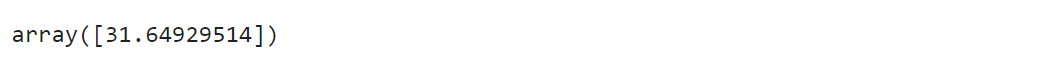• 数据清洗_缺失值处理
2021-09-08 17:22:45

原文链接：风一带你一起学习:数据清洗_缺失值处理

碎碎念念：大家好！我是风一、有人调侃做数据的、80%的时间都是花在数据清洗上、虽然有时很想反驳一下、但现实确实经常是如此，那么何为数据清洗？需要处理的数据主要又有哪些？那么就让我们开始吧！

所谓的清洗，是对数据集通过丢弃、填充、替换、去重等操作，达到去除异常、纠正错误、补足缺失的目的；在数据清洗的过程中、主要需要处理的有缺失值、异常值和重复值，以下篇幅为数据缺失值处理。

## 一、数据缺失的两种情况：

一种是行记录的缺失，这种情况又称为数据记录的丢失；另一种是数据列值的缺失，即由于各种原因导致的数据记录中某些列的值空缺 。

## 二、数据缺失的常用处理思路

缺失的数据记录通常都是无法找回的、那么针对数据列类型的缺失值数据、需如何处理?

• 直接丢弃：也就是删除带有缺失值的行记录(整行删除)或者列字段 (整列删除)，通过直接删除这种方法非常简单明了、处理起来也特别的方便，但删除就意味着会消减数据的维度、特征；尤其是存在大量的缺失值 或者 带有缺失值的数据记录存在着明显的数据分布规律或特征，这些场景下都不宜直接丢弃缺失值。

• 补全缺失值：相比直接删除而言、补全是更常用的缺失值处理方法。通过统计值(均值、中位数等)、预测值、专家补全等方法将缺失的数据补全、使数据达成一个完整的数据结构、对于后续的数据处理、分析和建模至关重要。

• 真值转化法：在很多场景中、是无法得知缺失值的分布规律，并且无法直接对缺失值做补全删除等处理；那么我们认为数据缺失也是一种规律，去承认缺失值的存在，比如用户性别字段、部分数据的性别是不全的，但又不能直接丢弃或者补全、那么就可以将其中的值分布状态转换为多个变量的真值状态（转换前：一个字段(值为 男、女、未知)； 转换后：多个字段 、男 （1 或 0）、 女 （1 或 0）、 未知 （1 或 0） ）。

• 不做任何处理：一般而言、这类缺失值不涉及到业务所比较关键的字段 或者不影响到后期的数据分析和建模应用，很多模型对于缺失值有容忍度或灵活的处理方法，比如：KNN、决策树等等。

## 三、代码实练

在缺失值的处理上、主要配合使用 sklearn.preprocessing 中 的 Imputer 类、Pandas 和 Numpy。

### 3.1.导入所需库并生成缺失数据

import pandas as pd
import numpy as np
# from sklearn.preprocessing import Imputer # 老版本

# Sklearn 专门处理缺失值的模块、官网地址：https://www.jianshu.com/p/2c59147e1df1
from sklearn.impute import SimpleImputer as Imputer

df = pd.DataFrame(np.random.randn(6, 4), columns = ['col1', 'col2', 'col3', 'col4'])
df.iloc[1:2, 1] = np.nan
df.iloc[4, 3] = np.nan

df


图片

### 3.2.判断缺失值

nan_all = df.isnull()  # 获取所有数据框中的 N 值（N值为 Trure）
print(nan_all)
print('*'*30)

col_all = df.isnull().sum()  # 统计每列缺失值的数量
print(col_all)
print('*'*30)

nan_col1 = df.isnull().all() # 获得全部为 NA 的列
print(nan_col1)
print('*'*30)

nan_col2 = df.isnull().any() # 获得含有 NA 的列
print(nan_col2)

    col1   col2   col3   col4
0  False  False  False  False
1  False   True  False  False
2  False  False  False  False
3  False  False  False  False
4  False  False  False   True
5  False  False  False  False
******************************
col1    0
col2    1
col3    0
col4    1
dtype: int64
******************************
col1    False
col2    False
col3    False
col4    False
dtype: bool
******************************
col1    False
col2     True
col3    False
col4     True
dtype: bool


### 3.3.丢弃缺失值

df2 = df.dropna(how = "any")   # 直接删除含有 NA 的行记录
print(df2)
print('*'*30)

df3 = df.copy()
df3.iloc[1:2,:] = np.nan  # 将第二行都设置为 NA
df3 = df3.dropna(how = "all")  # 删除全部为 NA 值的行记录
print(df3)

       col1      col2      col3      col4
0 -0.530849 -0.587055  0.754068  0.597813
2  0.146497  0.625747 -1.663007 -1.356476
3 -0.699932  0.390106  1.342162 -2.133165
5 -0.549286 -0.300173  1.889540  1.178008
******************************
col1      col2      col3      col4
0 -0.530849 -0.587055  0.754068  0.597813
2  0.146497  0.625747 -1.663007 -1.356476
3 -0.699932  0.390106  1.342162 -2.133165
4 -0.395517 -0.172803  0.233490       NaN
5 -0.549286 -0.300173  1.889540  1.178008

df


### 3.4.通过 sklearn 的数据预处理方法对缺失值进行处理

# 官网地址：https://scikit-learn.org/stable/modules/impute.html
nan_model = Imputer(missing_values = np.nan, strategy = 'mean') # 建立替换规则：将值为 NaN 的缺失值以均值做替换
nan_result = nan_model.fit_transform(df)
print(nan_result)

[[-0.53084907 -0.58705498  0.75406835  0.59781252]
[ 0.33872081 -0.00883559  2.19513579  1.79174735]
[ 0.14649702  0.62574696 -1.66300695 -1.35647607]
[-0.69993206  0.39010577  1.34216247 -2.13316465]
[-0.39551654 -0.17280315  0.23349011  0.01558536]
[-0.54928642 -0.30017256  1.88954049  1.17800765]]


注意事项：missing_values 默认空值 np.nan, 指定空值需 np.nan、直接写 ‘NaN’ 是不一样的、会报错。

strategy参数：

图片：

### 3.5.使用 Pandas 做缺失值处理

Pandas 对缺失值的处理方法是 df.fillna()，该方法中最主要的两个参数是 value 和 method。前者通过固定(或手动指定)的值替换缺失值，后者使用 pandas 提供默认方法替换缺失值。

''' DataFrame
def fillna(
self,
value=None, # 指定填充的值,可以是一个标量，或者字典等
method=None, # 指定填充的方法; [pad/ffill、 backfill/bfill、 None]
axis=None, # 指定轴
inplace=False, # 是否将结果赋值给原变量
limit=None,  # 限制每列替换缺失值的个数
):

'''

nan_result_pd1 = df.fillna(method = 'backfill')  # 使用后面的值替换缺失值
nan_result_pd1

       col1      col2      col3      col4
0 -0.530849 -0.587055  0.754068  0.597813
1  0.338721  0.625747  2.195136  1.791747
2  0.146497  0.625747 -1.663007 -1.356476
3 -0.699932  0.390106  1.342162 -2.133165
4 -0.395517 -0.172803  0.233490  1.178008
5 -0.549286 -0.300173  1.889540  1.178008


## 四、总结

在现实数据中、很容易出现缺失数据，尤其是数据维度特别大的情况下；那么针对不同类型、不同的业务数据当然会有不同的方法；有些可以确认无效数据可以直接删除、但往往大部分都是无法直接这样简单明了处理、需结合实际业务以及数据结构进行处理、这里风一就不一一展开详解。

说明：文章大部分篇幅来源于宋老师(宋天龙)所著书籍《Python数据分析与数据化运营》的读书笔记以及相应的学习扩展补充。

作者：在成长的风一
文章首发：公众号【在成长的风一】
未经允许禁止转载，需要转载请微信联系授权（微信号：Fy180920）python
更多相关内容
• 1.决策树缺失值处理 1.1对于训练模型，训练数据部分样本的部分特征值缺失的情况下，做法是：把该数据按比例分成三份数据。可以算出该特征的信息增益。 1.2对于预测数据，C4.5中采用的方法是：测试样本在该属性值上有...
• 缺失值处理 缺失值首先需要根据实际情况定义 可以采取直接删除法 有时候需要使用替换法或者插值法 常用的替换法有均值替换、前向、后向替换和常数替换 import pandas as pd import numpy as np import os os.getcwd...
• 入门级基础知识，本人学习整理笔记，对小白很友好，有兴趣的学习的朋友可以查阅。
• 主要介绍了python 缺失值处理的方法（Imputation），文中通过示例代码介绍的非常详细，对大家的学习或者工作具有一定的参考学习价值，需要的朋友们下面随着小编来一起学习学习吧
• 本文介绍通过拉格朗日插值法进行缺失值的填充： 先用一组简单的数据看一下拉格朗日插值法 from scipy.interpolate import lagrange import matplotlib.pyplot as plt import numpy as np %matplotlib inline plt....
• 首先是缺失值处理。 #读取数据 import pandas as pd filepath= 'F:/...'#本地文件目录 df= pd.read_csv(train,sep=',')#df数据格式为DataFrame 查看缺失值 查看每一特征是否缺失及缺失值数量可能影响着处理缺失值的...
• 当数据中存在缺失值时：比较科学的方法是做插值填补的方法，代码思路：从excel中导入数据，然后用matalab插值拟合，最终输入直接在excel表中修改。炒鸡简单实用 快捷，当然你也可以导入数据库文件，看个人需求！！...
• Python Pandas缺失值处理 在一些数据分析业务中，数据缺失是我们经常遇见的问题，缺失值会导致数据质量的下降，从而影响模型预测的准确性，这对于机器学习和数据挖掘影响尤为严重。因此妥善的处理缺失值能够使模型...

# Python Pandas缺失值处理

在一些数据分析业务中，数据缺失是我们经常遇见的问题，缺失值会导致数据质量的下降，从而影响模型预测的准确性，这对于机器学习和数据挖掘影响尤为严重。因此妥善的处理缺失值能够使模型预测更为准确和有效。

## 为什么会存在缺失值？

前面章节的示例中，我们遇到过很多 NaN 值，关于缺失值您可能会有很多疑问，数据为什么会丢失数据呢，又是从什么时候丢失的呢？通过下面场景，您会得到答案。

其实在很多时候，人们往往不愿意过多透露自己的信息。假如您正在对用户的产品体验做调查，在这个过程中您会发现，一些用户很乐意分享自己使用产品的体验，但他是不愿意透露自己的姓名和联系方式；还有一些用户愿意分享他们使用产品的全部经过，包括自己的姓名和联系方式。因此，总有一些数据会因为某些不可抗力的因素丢失，这种情况在现实生活中会经常遇到。

## 什么是稀疏数据？

稀疏数据，指的是在数据库或者数据集中存在大量缺失数据或者空值，我们把这样的数据集称为稀疏数据集。稀疏数据不是无效数据，只不过是信息不全而已，只要通过适当的方法就可以“变废为宝”。

稀疏数据的来源与产生原因有很多种，大致归为以下几种：

由于调查不当产生的稀疏数据；

由于天然限制产生的稀疏数据；

文本挖掘中产生的稀疏数据。

## 缺失值处理

那么 Pandas 是如何处理缺失值的呢，下面让我们一起看一下。

import pandas as pd
import numpy as np
df = pd.DataFrame(np.random.randn(5, 3), index=['a', 'c', 'e', 'f','h'])
df = df.reindex(['a', 'b', 'c', 'd', 'e', 'f', 'g', 'h'])
print(df)


输出结果：

          0         1         2
a  0.187208 -0.951407  0.316340
b       NaN       NaN       NaN
c -0.365741 -1.983977 -1.052170
d       NaN       NaN       NaN
e -1.024180  1.550515  0.317156
f -0.799921 -0.686590  1.383229
g       NaN       NaN       NaN
h -0.207958  0.426733 -0.325951


上述示例，通过使用 reindex（重构索引），我们创建了一个存在缺少值的 DataFrame 对象。

## 检查缺失值

为了使检测缺失值变得更容易，Pandas 提供了 isnull() 和 notnull() 两个函数，它们同时适用于 Series 和 DataFrame 对象。

import pandas as pd
import numpy as np
df = pd.DataFrame(np.random.randn(5, 3), index=['a', 'c', 'e', 'f','h'])
df = df.reindex(['a', 'b', 'c', 'd', 'e', 'f', 'g', 'h'])
print(df['noe'].isnull())


输出结果：

a    False
b     True
c    False
d     True
e    False
f    False
g     True
h    False
Name: 1, dtype: bool


notnull() 函数，使用示例：

import pandas as pd
import numpy as np
df = pd.DataFrame(np.random.randn(5, 3), index=['a', 'c', 'e', 'f','h'])
df = df.reindex(['a', 'b', 'c', 'd', 'e', 'f', 'g', 'h'])
print df['one'].notnull()


输出结果：

a     True
b    False
c     True
d    False
e     True
f     True
g    False
h     True
Name: 1, dtype: bool


## 缺失数据计算

计算缺失数据时，需要注意两点：首先数据求和时，将 NA 值视为 0 ，其次，如果要计算的数据为 NA，那么结果就是 NA。示例如下：

import pandas as pd
import numpy as np
df = pd.DataFrame(np.random.randn(5, 3), index=['a', 'c', 'e', 'f','h'],columns=['one', 'two', 'three'])
df = df.reindex(['a', 'b', 'c', 'd', 'e', 'f', 'g', 'h'])
print (df['one'].sum())
print()


输出结果：

3.4516595395128


## 清理并填充缺失值

Pandas 提供了多种方法来清除缺失值。fillna() 函数可以实现用非空数据“填充”NaN 值。

1) 用标量值替换NaN值

下列程序将 NaN 值 替换为了 0，如下所示：

import pandas as pd
import numpy as np
df = pd.DataFrame(np.random.randn(3, 3), index=['a', 'c', 'e'],columns=['one',
'two', 'three'])
df = df.reindex(['a', 'b', 'c'])
print(df)
#用 0 填充 NaN
print (df.fillna(0))


输出结果：

     one       two     three
a  1.497185 -0.703897 -0.050513
b       NaN       NaN       NaN
c  2.008315  1.342690 -0.255855

one       two     three
a  1.497185 -0.703897 -0.050513
b  0.000000  0.000000  0.000000
c  2.008315  1.342690 -0.255855


当然根据您自己的需求，您也可以用其他值进行填充。

2) 向前和向后填充NA

在前一节，我们介绍了 ffill() 向前填充和 bfill() 向后填充，使用这两个函数也可以处理 NA 值。示例如下：

import pandas as pd
import numpy as np
df = pd.DataFrame(np.random.randn(5, 3), index=['a', 'c', 'e', 'f','h'],columns=['one', 'two', 'three'])
df = df.reindex(['a', 'b', 'c', 'd', 'e', 'f', 'g', 'h'])
print df.fillna(method='ffill')


输出结果：

        one       two     three
a  0.871741  0.311057  0.091005
b  0.871741  0.311057  0.091005
c  0.107345 -0.662864  0.826716
d  0.107345 -0.662864  0.826716
e  1.630221  0.482504 -0.728767
f  1.283206 -0.145178  0.109155
g  1.283206 -0.145178  0.109155
h  0.222176  0.886768  0.347820


或者您也可以采用向后填充的方法。

3) 使用replace替换通用值

在某些情况下，您需要使用 replace() 将 DataFrame 中的通用值替换成特定值，这和使用 fillna() 函数替换 NaN 值是类似的。示例如下：

import pandas as pd
import numpy as np
df = pd.DataFrame({'one':[10,20,30,40,50,666], 'two':[99,0,30,40,50,60]})
#使用replace()方法
print (df.replace({99:10,666:60,0:20}))


输出结果：

   one  two
0   10   10
1   20   20
2   30   30
3   40   40
4   50   50
5   60   60


## 删除缺失值

如果想删除缺失值，那么使用 dropna() 函数与参数 axis 可以实现。在默认情况下，按照 axis=0 来按行处理，这意味着如果某一行中存在 NaN 值将会删除整行数据。示例如下：

import pandas as pd
import numpy as np
df = pd.DataFrame(np.random.randn(5, 3), index=['a', 'c', 'e', 'f','h'],columns=['one', 'two', 'three'])
df = df.reindex(['a', 'b', 'c', 'd', 'e', 'f', 'g', 'h'])
print(df)
#删除缺失值
print (df.dropna())


输出结果：

        one       two     three
a -2.025435  0.617616  0.862096
b       NaN       NaN       NaN
c -1.710705  1.780539 -2.313227
d       NaN       NaN       NaN
e -2.347188 -0.498857 -1.070605
f -0.159588  1.205773 -0.046752
g       NaN       NaN       NaN
h -0.549372 -1.740350  0.444356

one       two     three
a -2.025435  0.617616  0.862096
c -1.710705  1.780539 -2.313227
e -2.347188 -0.498857 -1.070605
f -0.159588  1.205773 -0.046752
h -0.549372 -1.740350  0.444356


axis = 1 表示按列处理，处理结果是一个空的 DataFrame 对象。

展开全文• 因近期进行时间序列分析时遇到了数据预处理中的缺失值处理问题，其中日期缺失和填充在网上没有找到较好较全资料，耗费了我一晚上工作时间，所以下面我对这次时间序列缺失值处理学习做了以下小结以供之后同行们参考...
• 主要介绍缺失值的定义、产生与处理，对缺失值处理时的一些影响
• 但是如果缺失值占比比较大，这种直接删除缺失值处理方法就会丢失重要信息。 直接删除法处理缺失值时，需要检测样本总体中缺失值的个数。Python中统计缺失值的方法如下（下面结合具体数据集，直接上代码）： import...
• R语言处理缺失值处理数据过程中，避免不了会产生一些缺失值，如未填写数据或者编码错误等原因，用NA表示缺失值。在R语言中，is.na()函数可以判断元素是否是缺失值，从而返回逻辑值(TRUE/FALSE)，所以该函数将会...r语言
• 缺失值处理 数据缺失的原因 缺失值的产生的原因多种多样，主要分为机械原因和人为原因。机械原因是由于机械原因导致的数据收集或保存的失败造成的数据缺失，比如数据存储的失败，存储器损坏，机械故障导致某段时间...

# 缺失值处理

## 数据缺失的原因

缺失值的产生的原因多种多样，主要分为机械原因和人为原因。机械原因是由于机械原因导致的数据收集或保存的失败造成的数据缺失，比如数据存储的失败，存储器损坏，机械故障导致某段时间数据未能收集（对于定时数据采集而言）。人为原因是由于人的主观失误、历史局限或有意隐瞒造成的数据缺失，比如，在市场调查中被访人拒绝透露相关问题的答案，或者回答的问题是无效的，数据录入人员失误漏录了数据

## 数据缺失的类型

将数据集中不含缺失值的变量称为 完全变量 ，数据集中含有缺失值的变量称为 不完全变量

### 完全随机缺失（MCAR）

指的是数据的缺失是随机的，不依赖于任何不完全变量或完全变量，不影响样本的无偏性，如家庭地址缺失

### 随机缺失（MAR）

指的是数据的缺失不是完全随机的，即该类数据的缺失依赖于其他完全变量，如财务数据缺失情况与企业的大小有关

### 非完全随机缺失（MNAR）

指的是数据的缺失依赖于不完全变量自身，如高收入人群不愿意提供家庭收入

## 数据缺失的处理方法

### 一、不处理

有一些模型自身能够处理数据缺失的情况（如随机森林、xgboost），在这种情况下不需要对缺失数据做任何的处理，这种做法的缺点是在模型的选择上有局限

### 二、删除

import pandas as pd
import numpy as np
from sklearn.impute import KNNImputer
from sklearn.ensemble import RandomForestClassifier

df = pd.read_csv('person.csv')
df#### 删除有缺失数据的样本

df = pd.read_csv('person.csv')
df = df.dropna(how='any')
df#### 删除有过多缺失数据的特征

df = pd.read_csv('person.csv')
df = df.drop(['height','weight'], axis=1)
df### 三、填充

#### 固定值填充

df = pd.read_csv('person.csv')
df['sex'] = df['sex'].fillna('female')
df#### 均值填充

df = pd.read_csv('person.csv')
df['height'] = df['height'].fillna(df['height'].mean())
df#### 中位数填充

df = pd.read_csv('person.csv')
df['height'] = df['height'].fillna(df['height'].median())
df#### 众数填充

df = pd.read_csv('person.csv')
df['age'] = df['age'].fillna(df['age'].mode()) # The mode is the value that appears most often
df#### 用上一个值填充

df = pd.read_csv('person.csv')
df['height'] = df['height'].ffill()
df#### 用下一个值填充

df = pd.read_csv('person.csv')
df['weight'] = df['weight'].bfill()
df#### 随机数填充

df = pd.read_csv('person.csv')
r = np.random.randint(2) % 2 # [0,2)
df['sex'] = df['sex'].fillna('female' if r == 1  else 'male')
df#### 插值法填充

使用插值法可以计算缺失值的估计值，所谓的插值法就是通过两点 ( x 0 , y 0 ) (x_0,y_0) ( x 1 , y 1 ) (x_1,y_1) 估计中间点的值，假设 y = f ( x ) y=f(x) 是一条直线，通过已知的两点来计算函数 f ( x ) f(x) ，然后只要知道 x x 就能求出 y y ，以此方法来估计缺失值。当然我们也可以假设 f ( x ) f(x) 不是直线，而是其他函数

df = pd.read_csv('person.csv')
# 默认为线性插值，即在两个数据点之间连接直线，计算给定的插值点在直线上的值作为插值结果
df['age'] = df['age'].interpolate() # nan=(24+26)/2=25
df#### KNN 填充

df = pd.read_csv('person.csv')
# 标签编码
df['sex'] = df['sex'].map({'female': 1
,'male': 0})
df# 可以使用交叉验证来选择k值
imputer = KNNImputer(n_neighbors=1) # 默认每个邻域中的所有点的权重均相等
df.iloc[:,1:] = imputer.fit_transform(df.iloc[:,1:])
df#### 建模预测

将缺失的属性作为预测目标来预测，将数据集按照是否含有特定属性的缺失值分为两类，利用现有的机器学习算法对预测数据集的缺失值进行预测。该方法的根本的缺陷是如果其他属性和缺失属性无关，则预测的结果毫无意义；但是若预测结果相当准确，则说明这个缺失属性是没必要纳入数据集中的；一般的情况是介于两者之间

下面的案例中将选择 sex 特征作为预测目标，采用随机森林进行拟合得到预测值，其他缺失特征采用均值进行填充

# 读入数据

# 标签编码
df['sex'] = df['sex'].map({'female': 1
,'male': 0})

# 分离特征和标签
feature = df.iloc[:,2:]
df.iloc[:,2:] = feature.fillna(feature.mean()) # 其他缺失特征采用均值进行填充
feature = df.iloc[:,2:] # age height weight
label = df.iloc[:,1] # sex

print(feature)
print(label)# 将sex非空划分为训练集
sex_is_null = df['sex'].isnull()
train_indices = sex_is_null == False
X_train = feature[train_indices]
y_train = label[train_indices]
# 将sex为空划分为测试集
X_test = feature[sex_is_null]

print(X_train)
print(y_train)
print(X_test)# 构建模型，训练并预测
rf = RandomForestClassifier(n_estimators=10, class_weight='balanced', random_state=1)
rf.fit(X_train, y_train)
predictions = rf.predict(X_test)
df.loc[sex_is_null,'sex'] = predictions
df#### 高维映射

将属性映射到高维空间，采用独热编码（one-hot）技术对属性进行编码。将包含 K 个离散取值范围的属性值扩展为 K+1 个属性值，若该属性值缺失，则扩展后的第 K+1 个属性值置为1。这种做法是最精确的做法，保留了所有的信息，也未添加任何额外信息，若预处理时把所有的变量都这样处理，会大大增加数据的维度。这样做的好处是完整保留了原始数据的全部信息，不用考虑缺失值；缺点是计算量大大提升，且只有在样本量非常大的时候效果才好

df = pd.read_csv('person.csv')
dummy_sex = pd.get_dummies(df['sex'], prefix='sex', dummy_na=True)
df = pd.concat([df, dummy_sex], axis=1)
df = df.drop('sex', axis=1)
df#### 热卡填充

对于一个包含空值的对象，热卡填充法（Hot deck imputation）在完整数据中找到一个与它最相似的对象，然后用这个相似对象的值来进行填充。不同的问题可能会选用不同的标准来对相似进行判定。该方法概念上很简单，且利用了数据间的关系来进行空值估计，但缺点在于难以定义相似标准，主观因素较多

#### 极大似然估计

在缺失类型为随机缺失的条件下，假设模型对于完整的样本是正确的，那么通过观测数据的边际分布可以对未知参数进行极大似然估计（Little and Rubin）。这种方法也被称为忽略缺失值的极大似然估计，对于极大似然的参数估计实际中常采用的计算方法是期望值最大化（Expectation Maximization，EM）。该方法比删除个案和单值插补更有吸引力，它一个重要前提：适用于大样本。有效样本的数量足够以保证极大似然估计值是渐近无偏的并服从正态分布。但是这种方法可能会陷入局部极值，收敛速度也不是很快，并且计算很复杂

#### 多重插补

多值插补的思想来源于贝叶斯估计，认为待插补的值是随机的，它的值来自于已观测到的值。具体实践上通常是估计出待插补的值，然后再加上不同的噪声，形成多组可选插补值。根据某种选择依据，选取最合适的插补值

## 总结

当我们决定采用何种缺失值处理方式时，要综合考虑数据缺失的原因、数据缺失的类型、数据缺失的处理方法的特点、样本量等因素

本文到此结束，后续将会不断更新，如果发现上述有误，请各位大佬及时指正！如果觉得写得还可以，欢迎点赞收藏加关注，谢谢！

展开全文机器学习 数据预处理
• 该Eviews文件对应我的博客（平衡面板数据中的缺失值可以存在吗？https://blog.csdn.net/zhouxiaojieaaa/article/details/110999819）
• 5、将姓名的缺失值进行填充 6、将清洗好的数据保存到指定excel中 原始数据： 最后输出数据： 处理步骤： 1、读取数据： 2、删除全是空值的列 3、删除全是空值的行 4、将分数列值为NAN（空值）的填充为0分 5、...
• 该Eviews文件对应我的博客-（平衡面板数据中的缺失值可以存在吗？https://www.jianshu.com/p/39d2f529237b）
• 缺失值处理 信息系统往往是不完备的，经常会有数据缺失。产生数据缺失一般有两种原因，第一种是有些信息暂时无法获取，例如一个单身人士的配偶或者一个儿童的收入等；第二种是有些信息被遗漏或者错误的被处理了。 ...

## 缺失值处理

信息系统往往是不完备的，经常会有数据缺失。产生数据缺失一般有两种原因，第一种是有些信息暂时无法获取，例如一个单身人士的配偶或者一个儿童的收入等；第二种是有些信息被遗漏或者错误的被处理了。

数据缺失是不可避免的，我们经常要做缺失数据的处理，处理缺失数据通常有三种方法：
1、第一种是不处理，例如单身人士的配偶，不存在是很正常的。
2、第二种是删除缺失值所对应的行，这种方法在样本数据较少时，谨慎使用。
3、第三种是数据补齐，使用规则或模型，对缺失的数据进行补齐。

下面使用代码一一实现这三种方法：
示例数据：数据中“年龄”和“工资”两列分别有一个缺失值。

### 删除缺失值所在的行：

操作非常简单，直接使用DataFrame模块中的dropna函数即可。

import pandas as pd
'../form/缺失值.csv')
#直接删除缺失值


执行代码，可以得到删除后的数据：### 均值/众数/中值填充

在sklearn模块中，使用Imputer函数对数据中的缺失值进行填充。

sklearn.preprocessing.Imputer(strategy = ‘mean’)

参数说明
strategy数据填充方式，默认使用均值填充，可选：median和most_frequent
from sklearn.impute import SimpleImputer
#使用均值填充缺失值
simpleImputer = SimpleImputer(strategy = 'mean')
data['年龄_imputer'] = simpleImputer.fit_transform(data[['年龄']])
data['工资_imputer'] = simpleImputer.fit_transform(data[['工资']])


执行代码，可以得到填充后的数据：### 模型填充

训练模型使用有监督的方式填充缺失值

步骤：
1、确定要填充哪列的缺失值

2、把要处理的列作为目标列，其他列作为特征列。清除特征列中的缺失值

3、把目标列中的缺失值过滤出来，作为预测数据，其他数据作为训练数据进行建模

4、对训练数据进行特征处理

5、训练模型，对缺失值进行预测

下面按照步骤对年龄进行填充。

#先处理年龄的缺失值
data_predict_年龄 = data.dropna(subset = ['工资'])
#找出剩下数据中，年龄缺失值所在位置
NA_index = data_predict_年龄.年龄.isna()
#获取训练数据和预测数据
data_predict_年龄_fit = data_predict_年龄[~NA_index]
data_predict_年龄_predict = data_predict_年龄[NA_index]


开始进行线性回归建模

from sklearn.preprocessing import OneHotEncoder
#对训练数据进行特征处理
oneHotEncoder = OneHotEncoder()
oneHotData_fit = oneHotEncoder.fit_transform(
data_predict_年龄_fit[['国家','购买']])


将独热编码所得的数据和工资数据进行合并
独热编码

from scipy.sparse import hstack
x_fit = hstack([
oneHotData_fit,
data_predict_年龄_fit.工资.values.reshape(-1,1)])
y_fit = data_predict_年龄_fit['年龄']


训练线性回归模型，对缺失值进行预测

from sklearn.linear_model import LinearRegression
linearRegression = LinearRegression()
linearRegression.fit(x_fit,y_fit)
#处理要预测的数据的特征
oneHotData_predict = oneHotEncoder.transform(
data_predict_年龄_predict[['国家','购买']])

x_predict = hstack([
oneHotData_predict,
data_predict_年龄_predict.工资.values.reshape(-1,1)
])
#预测缺失值
linearRegression.predict(x_predict)


执行代码，可以看到，通过模型预测的年龄缺失值为31.65，而通过均值填充的年龄缺失值为38.78，很明显模型预测的年龄缺失值更加合理，但是工作量比较大。展开全文机器学习 数据挖掘 python
• 主要给大家介绍了关于Python Pandas对缺失值处理方法，文中通过示例代码介绍的非常详细，对大家学习或者使用Python Pandas具有一定的参考学习价值，需要的朋友们下面来一起学习学习吧
• 处理缺失值的意义 没有高质量的数据，就没有高质量的数据挖掘结果，数据值缺失是数据分析中经常遇到的问题之一。当缺失比例很小时，可直接对缺失记录进行舍弃或进行手工处理。但在实际数据中，往往缺失数据占有相当...
• ## 缺失值处理python实现

万次阅读 多人点赞 2019-02-22 14:10:29
缺失值的处理 由于各种原因，我们使用的数据集或多或少地存在着缺失值。如果直接删除缺失值，会丢失其他有价值的数据，以下是处理缺失值的几种方法...但是如果缺失值占比上升，这种缺失值处理方法误差就很大了。 &a均值填充
• 我们的数据样本中经常会出现缺失值的情况，那么在数据清洗的过程中，怎么处理缺失值？对于缺失值有三类处理方法： 删除 填充 不处理 1.删除   对于缺失情况很严重的变量（缺失比例0.8-0.9以上），一般采用直接...python
• 今天我们来看看数据预处理中一个有趣的问题：数据清理中，如何处理缺失值。在我们探讨问题之前，我们一起回顾一些基本术语，帮助我们了解为什么需要关注缺失值。目录数据清洗简介填补缺失值的重要性缺失...算法 人工智能 大数据 python 机器学习
• 缺失值是指粗糙数据中由于缺少信息而造成的数据的删失或截断。它指的是现有数据集中某个或某些属性的值是不完全的。 值得注意的是，这里所说的缺失值，不仅包括数据库中的NULL值，也包括用于表示数值缺失的特殊数值...sql 数据库 神经网络 数据挖掘 python...